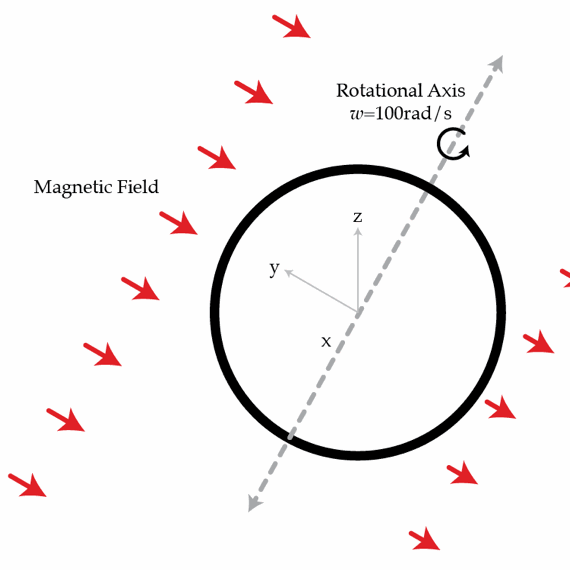# Nonstandard RL circuit

A thin ring with resistance $R=10~\Omega$ and self inductance $L=0.2~\mbox{H}$ rotates with constant angular speed $\omega=100~\mbox{rad/s}$ in an external uniform magnetic field perpendicular to the rotation axis. As a result, the magnetic flux created by the external field varies with time as $\Phi(t)=\Phi_{0}\cos(\omega t) \quad \textrm{where} \quad \Phi_{0}=0.1~\mbox{Wb} .$ Because of the resistance of the ring, energy is being continuously dissipated in the system. What average power $\bar{P}$ in Watts must develop the external forces to keep the ring rotating at constant angular speed $\omega$ (on average).×

Problem Loading...

Note Loading...

Set Loading...